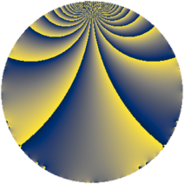# Properties

 Label 3528.1.biLevel $3528$ Weight $1$ Character orbit 3528.bi Rep. character $\chi_{3528}(1157,\cdot)$ Character field $\Q(\zeta_{6})$ Dimension $8$ Newform subspaces $1$ Sturm bound $672$ Trace bound $0$

# Related objects

## Defining parameters

 Level: $$N$$ $$=$$ $$3528 = 2^{3} \cdot 3^{2} \cdot 7^{2}$$ Weight: $$k$$ $$=$$ $$1$$ Character orbit: $$[\chi]$$ $$=$$ 3528.bi (of order $$6$$ and degree $$2$$) Character conductor: $$\operatorname{cond}(\chi)$$ $$=$$ $$504$$ Character field: $$\Q(\zeta_{6})$$ Newform subspaces: $$1$$ Sturm bound: $$672$$ Trace bound: $$0$$

## Dimensions

The following table gives the dimensions of various subspaces of $$M_{1}(3528, [\chi])$$.

Total New Old
Modular forms 40 24 16
Cusp forms 8 8 0
Eisenstein series 32 16 16

The following table gives the dimensions of subspaces with specified projective image type.

$$D_n$$ $$A_4$$ $$S_4$$ $$A_5$$
Dimension 8 0 0 0

## Trace form

 $$8q - 8q^{4} + O(q^{10})$$ $$8q - 8q^{4} - 8q^{15} + 8q^{16} - 4q^{18} + 12q^{23} - 4q^{25} + 4q^{30} - 8q^{39} + 12q^{50} + 4q^{57} + 8q^{60} - 8q^{64} + 4q^{72} + 8q^{78} + 4q^{81} - 12q^{92} + O(q^{100})$$

## Decomposition of $$S_{1}^{\mathrm{new}}(3528, [\chi])$$ into newform subspaces

Label Dim. $$A$$ Field Image CM RM Traces $q$-expansion
$$a_2$$ $$a_3$$ $$a_5$$ $$a_7$$
3528.1.bi.a $$8$$ $$1.761$$ $$\Q(\zeta_{24})$$ $$D_{12}$$ $$\Q(\sqrt{-14})$$ None $$0$$ $$0$$ $$0$$ $$0$$ $$q-\zeta_{24}^{6}q^{2}-\zeta_{24}^{7}q^{3}-q^{4}+(-\zeta_{24}^{3}+\cdots)q^{5}+\cdots$$